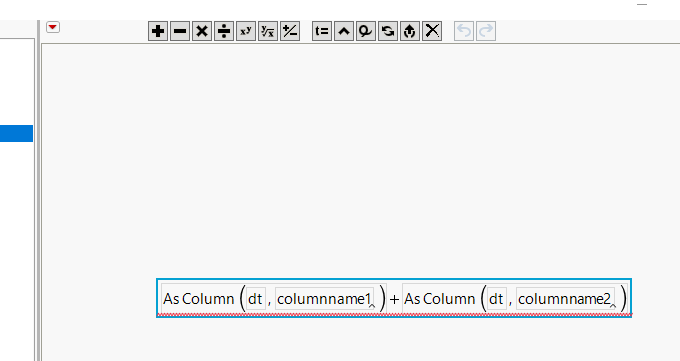Our World Statistics Day conversations have been a great reminder of how much statistics can inform our lives. Do you have an example of how statistics has made a difference in your life? Share your story with the Community!
Choose Language Hide Translation Bar
Highlighted

## call a column by referring to variable

A complete newbie here,

could not find the solution in older posts,

I have created a variable containing an existing column name. I want to create a new column, were the data from two other columns is summed (calling the columns by variables). the usage of variable is for later use in a for loop.

Thanks for helping!

for the below code:

``````dt = Current Data Table();

columnname1 = Column( dt, 1 ) << Get Name;
columnname2 = Column( dt, 2 ) << Get Name;

dt << New Column( "new_" || columnname1, Numeric , continuous, formula(column(dt, columnname1)+column(dt, columnname2)));``````

I get the following error:

Column new_Column 1 2 Formula Interrupted
Cannot convert argument to a number [or matrix] 1 times At rows: 2 Operation: Add, Column( dt, columnname1 ) + /*###*/Column( dt, columnname2 ) /*###*/
Formula evaluation errors have been ignored

Column( "new_Column 1 2" )

1 ACCEPTED SOLUTION

Accepted Solutions
HighlightedDavid_Burnham
Super User

## Re: call a column by referring to variable

You've already created variables that reference the columns.  You can use those in your formula, but you need to use the AsColumn function:

``formula( AsColumn(columnname1) + AsColumn(columnname2) )``
-Dave
4 REPLIES 4
HighlightedDavid_Burnham
Super User

## Re: call a column by referring to variable

You've already created variables that reference the columns.  You can use those in your formula, but you need to use the AsColumn function:

``formula( AsColumn(columnname1) + AsColumn(columnname2) )``
-Dave
Highlighted

## Re: call a column by referring to variable

You can get it to work, with

``````Names Default To Here( 1 );
dt = Open( "\$SAMPLE_DATA/big class.jmp" );
columnname1 = Column( dt, 4 ) << Get Name;
columnname2 = Column( dt, 5 ) << Get Name;

dt << New Column( "new_" || columnname1,
Numeric,
continuous,
formula(
As Column( dt, columnname1 ) + As Column( dt, columnname2 )
)
);``````

However, there is a potential problem with this.  The stored formula is:This formula will continue to work, as long as your JMP session

1. Has the variables, dt, columnname1 and columnname2 defined.  What happens if you save the data table, shutdown JMP, and then tomorrow you come in and startup JMP, and open the data table.  The formula will not find those variables, and the formula will fail.
2. Within the session, the variables, dt, columnname1 and columnname2, do not change their value.  So, if you create this column, and later on in your script, you happen to change the value of variable columnname1, since formula columns in a JMP data table are dynamic, the formula will rerun when changes to the data table take place, JMP will use the new value of the variable, and will create new values for the formula column.

What you really need to do, is to use a method that creates the actual JSL syntax for the formula.  In the above example, what you really want is for the formula to be

``:height + :weight``

The JSL below is one way to do this.  It uses the Substitute() function to implant the actual column names into the formula.

``````Names Default To Here( 1 );
dt = Open( "\$SAMPLE_DATA/big class.jmp" );
columnname1 = Column( dt, 4 ) << Get Name;
columnname2 = Column( dt, 5 ) << Get Name;

Eval(
Substitute(
Expr(
dt << New Column( "new_" || columnname1,
Numeric,
continuous,
formula( __c1__ + __c2__ )
)
),
Expr( __c1__ ), Parse( ":" || columnname1 ),
Expr( __c2__ ), Parse( ":" || columnname2 )
)
);``````
Jim
Highlighted

## Re: call a column by referring to variableDavid_Burnham